CAT  >  Practice Questions Level 1: Pie Chart

# Practice Questions Level 1: Pie Chart - Notes | Study Level-wise Practice Questions for CAT Preparation - CAT

 1 Crore+ students have signed up on EduRev. Have you?

This EduRev document offers 20 Multiple Choice Questions (MCQs) from the topic Pie Chart (Level - 1). These questions are of Level - 1 difficulty and will assist you in the preparation of CAT & other MBA exams. You can practice/attempt these CAT Multiple Choice Questions (MCQs) and check the explanations for a better understanding of the topic.

Question for Practice Questions Level 1: Pie Chart
Try yourself:Directions: Study the given information carefully and answer the following question.
The following pie chart shows the distribution of employees (in %) working in different departments of a company.
Total number of employees = 2400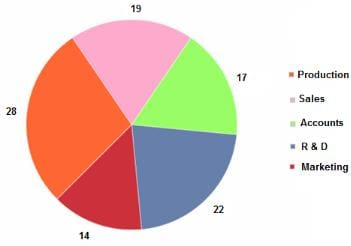What is the total number of employees working in Accounts, Production and Marketing?

Question for Practice Questions Level 1: Pie Chart
Try yourself:Directions: Study the following information and answer the question that follows.
The percentage of employees in various departments in company A is as shown below.
There are 1800 employees in total.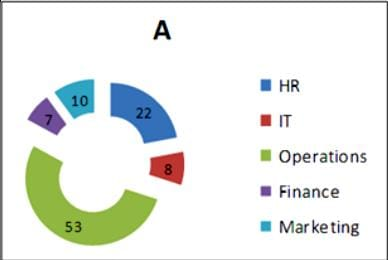How many employees are there in marketing and finance departments?

Question for Practice Questions Level 1: Pie Chart
Try yourself:Directions: Answer the given question based on the following information.
The pie chart below shows the expenditure of a company on different sectors.
Total budget of the company = Rs. 1660 crore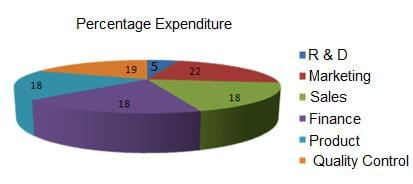What is the average expenditure on marketing and sales?

Question for Practice Questions Level 1: Pie Chart
Try yourself:Directions: Answer the given question based on the following information.
The pie chart below shows the expenditure of a company on different sectors.
Total budget of the company = Rs. 1660 croreWhat is the total expenditure on marketing, sales and finance?

Question for Practice Questions Level 1: Pie Chart
Try yourself:Directions: Answer the given question based on the following information.
The pie chart below shows the expenditure of a company on different sectors.
Total budget of the company = Rs. 1660 croreWhat is the expenditure on R & D?

Question for Practice Questions Level 1: Pie Chart
Try yourself:Directions: Study the following pie charts and answer the question.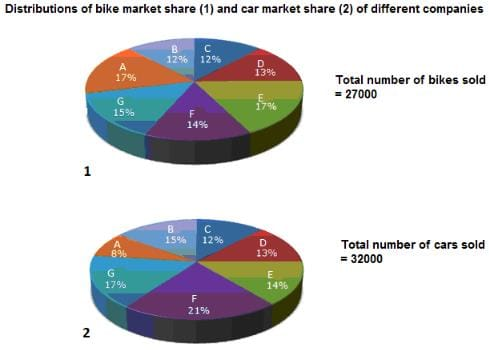What is the total number of bikes and cars sold by company F?

Question for Practice Questions Level 1: Pie Chart
Try yourself:Directions: Study the following pie charts and answer the question.Total number of bikes sold by companies A, B and C is

Question for Practice Questions Level 1: Pie Chart
Try yourself:Directions: Study the following pie charts and answer the question.What is the ratio of the number of bikes to that of cars sold by company E?

Question for Practice Questions Level 1: Pie Chart
Try yourself:Directions: Study the following pie charts and answer the question.What is the percentage of bikes sold by company G to the total sale of bikes and cars by all companies?

Question for Practice Questions Level 1: Pie Chart
Try yourself:Directions: The question is based on the following graph.
Pattern of Runs Scored by Lara in a Series of Six Test Matches.
Note : Runs scored are calculated to nearest whole number.
Total Runs = 664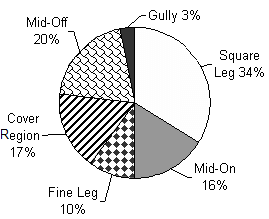From the pattern of the runs scored, Lara is strongest in which of the following regions?

Question for Practice Questions Level 1: Pie Chart
Try yourself:Directions: The question is based on the following graph.
Pattern of Runs Scored by Lara in a Series of Six Test Matches.
Note : Runs scored are calculated to nearest whole number.
Total Runs = 664Runs scored in the gully region form what percentage of runs scored in the fine leg region?

Question for Practice Questions Level 1: Pie Chart
Try yourself:Directions: The question is based on the following graph.
Pattern of Runs Scored by Lara in a Series of Six Test Matches.
Note : Runs scored are calculated to nearest whole number.
Total Runs = 664Had Lara struck four more 4's in the cover region(provided all other percentages remain same), the percentage of runs he scored in the cover region as a percentage of total runs would have been

Question for Practice Questions Level 1: Pie Chart
Try yourself:Directions: The question is based on the following graph.
Pattern of Runs Scored by Lara in a Series of Six Test Matches.
Note : Runs scored are calculated to nearest whole number.
Total Runs = 664If Lara's average before the beginning of the first test of the series was 50.84 in 90 innings and he played a total of 11 innings in this series, the average score per innings of Lara at the end of the series was

Question for Practice Questions Level 1: Pie Chart
Try yourself:Directions: The following pie charts give information about the percentage distribution of total notebooks of different brands sold by a book store during different months and the percentage distribution of profit earned from the sale of each brand: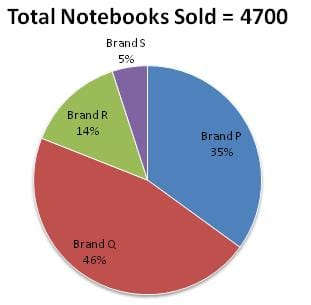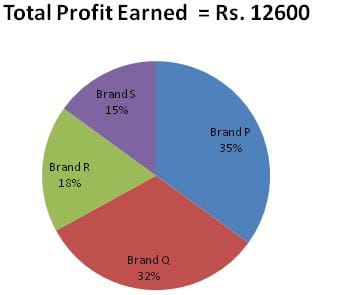If the book store offered 20% discount on brand P notebooks and earned 20% profit, then what was the marked price per notebook of brand P?

Question for Practice Questions Level 1: Pie Chart
Try yourself:Directions: The following pie charts give information about the percentage distribution of total notebooks of different brands sold by a book store during different months and the percentage distribution of profit earned from the sale of each brand:The profit earned per notebook of brand Q is what percent of the profit earned per notebook of brand R?

Question for Practice Questions Level 1: Pie Chart
Try yourself:Directions: The following pie charts give information about the percentage distribution of total notebooks of different brands sold by a book store during different months and the percentage distribution of profit earned from the sale of each brand:If each notebook of brand S was sold at a 25% profit after offering a 30% discount, then what was its marked price?

Question for Practice Questions Level 1: Pie Chart
Try yourself:Directions: Study the given pie chart carefully and answer the following question.
The following pie chart shows the total number of cars sold by eight car companies during the year 2016: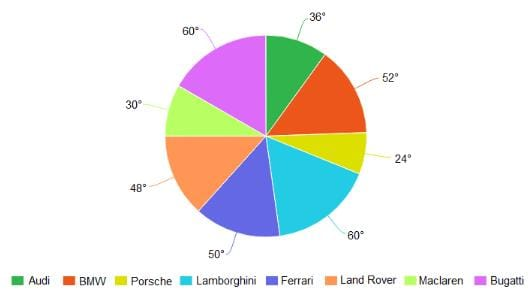If the total number of cars sold by Ferrari was 44,000, then what was the difference between the total number of cars sold by Bugatti and that sold by Porsche?

Question for Practice Questions Level 1: Pie Chart
Try yourself:Directions: Study the given pie chart carefully and answer the following question.
The following pie chart shows the total number of cars sold by eight car companies during the year 2016:What is the ratio of 20% of the total cars sold by Land Rover to 50% of the total cars sold by Audi?

Question for Practice Questions Level 1: Pie Chart
Try yourself:Directions: Study the given pie chart carefully and answer the following question.
The following pie chart shows the total number of cars sold by eight car companies during the year 2016:If the number of cars sold by Maclaren is 36,000, then what is the sum of two-thirds of the cars sold by BMW and nine-tenths of the cars sold by Lamborghini?

Question for Practice Questions Level 1: Pie Chart
Try yourself:Directions: Study the given pie chart carefully and answer the following question.
The following pie chart shows the total number of cars sold by eight car companies during the year 2016:What percent more cars were sold by Ferrari and Land Rover together than sold by Porsche and Lamborghini together?

The document Practice Questions Level 1: Pie Chart - Notes | Study Level-wise Practice Questions for CAT Preparation - CAT is a part of the CAT Course Level-wise Practice Questions for CAT Preparation.
All you need of CAT at this link: CAT

## Level-wise Practice Questions for CAT Preparation

277 docs
 Use Code STAYHOME200 and get INR 200 additional OFF

## Level-wise Practice Questions for CAT Preparation

277 docs

### How to Prepare for CAT

Read our guide to prepare for CAT which is created by Toppers & the best Teachers

Track your progress, build streaks, highlight & save important lessons and more!

,

,

,

,

,

,

,

,

,

,

,

,

,

,

,

,

,

,

,

,

,

;